Question

# F. pH of Buffer after the addition of the Strong Base 1. Calculate the expected pH...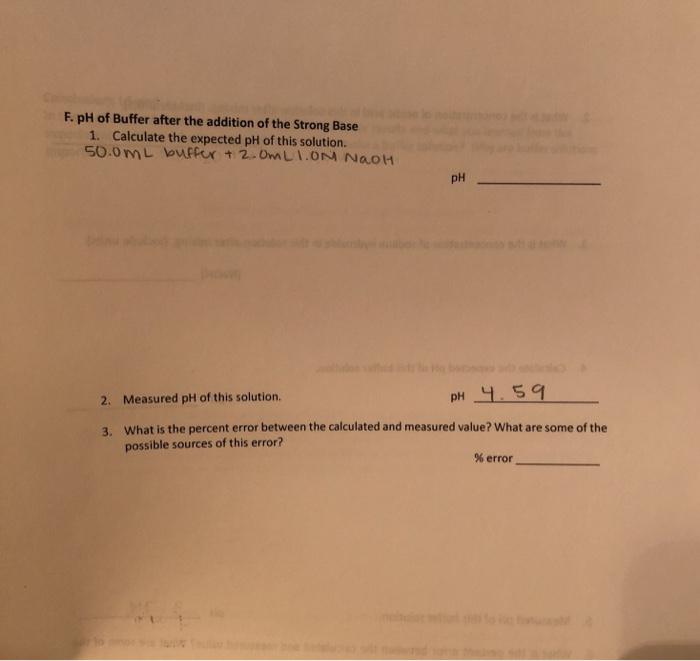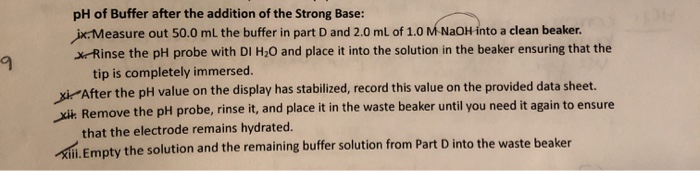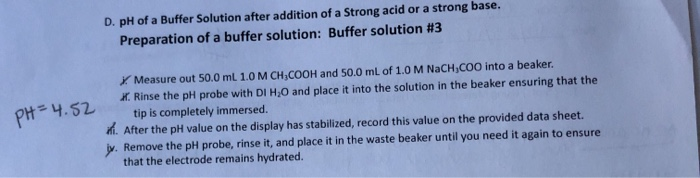F. pH of Buffer after the addition of the Strong Base 1. Calculate the expected pH of this solution. 50.0 mL buffer + 2.0mLION NAOH pH 2. Measured pH of this solution. pH 4.59 3. What is the percent error between the calculated and measured value? What are some of the possible sources of this error? % error
pH of Buffer after the addition of the Strong Base: ix. Measure out 50.0 ml the buffer in part D and 2.0 mL of 1.0 M NaOH into a clean beaker. x Rinse the pH probe with DI H20 and place it into the solution in the beaker ensuring that the tip is completely immersed. xiAfter the pH value on the display has stabilized, record this value on the provided data sheet. xit. Remove the pH probe, rinse it, and place it in the waste beaker until you need it again to ensure that the electrode remains hydrated. Empty the solution and the remaining buffer solution from Part D into the waste beaker
D. pH of a Buffer Solution after addition of a Strong acid or a strong base. Preparation of a buffer solution: Buffer solution #3 PH=4.52 X Measure out 50.0 ml 1.0 M CH,COOH and 50.0 mL of 1.0 M NaCH,COO into a beaker. X. Rinse the pH probe with DIH,O and place it into the solution in the beaker ensuring that the tip is completely immersed. m. After the pH value on the display has stabilized, record this value on the provided data sheet. J. Remove the pH probe, rinse it, and place it in the waste beaker until you need it again to ensure that the electrode remains hydrated.

F) 50 ml buffer contains 25 ml CH3COOH,25 ml CH3COONa.

No of mol of CH3COOH = 25*1 = 25 mmol

No of mol of NaOH = 25*1 = 25 mmol

pH of acidic buffer = pka + log(CH3COONa+NaOH/CH3COOH-NaOH)

pka of CH3COOH = 4.74

No of mol of NaOH added = 2*1 = 2 mmol

pH = 4.74+log((25+2)/(25-2))

pH = 4.81

Theoretical pH = 4.81

2) Measured (Experimental) pH = 4.59

Theoretical pH = 4.81

%error = (Theoretical- Experimental)/Theoretical*100

= (4.81-4.59)/4.81*100

= 4.57%

#### Earn Coins

Coins can be redeemed for fabulous gifts.

Similar Homework Help Questions
• ### The buffer solution is made of 50ml of 1.0M CH3COOH and 50ml of 1.0M NaCH3COO. Please...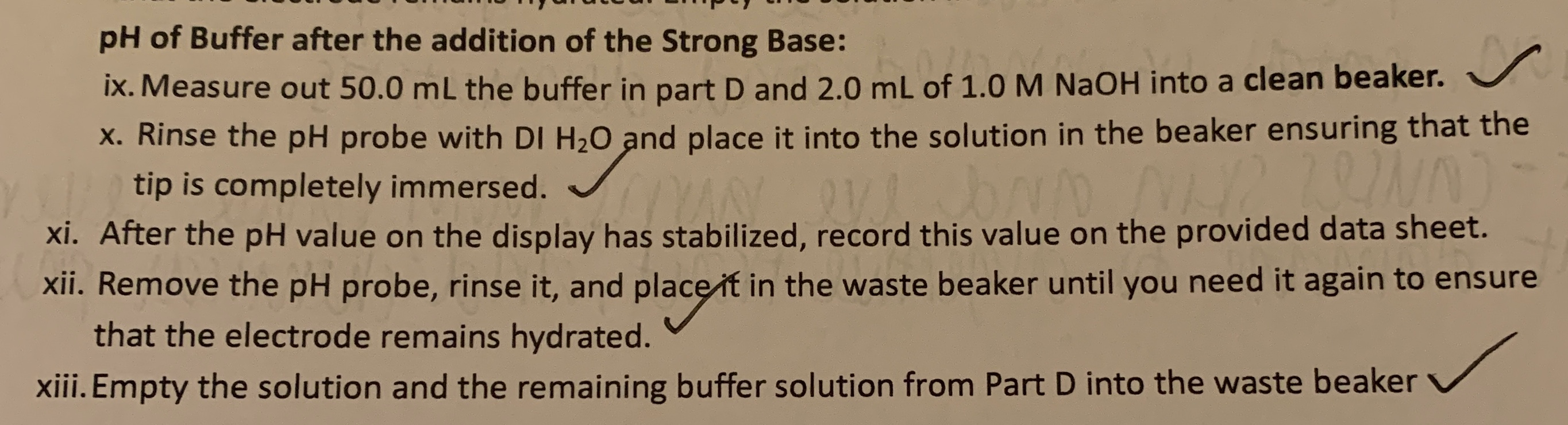The buffer solution is made of 50ml of 1.0M CH3COOH and 50ml of 1.0M NaCH3COO. Please find the expected pH of the buffer after the addition of the strong base, and the percent error between the expected pH and the measured pH of the solution. pH of Buffer after the addition of the Strong Base: ix. Measure out 50.0 mL the buffer in part D and 2.0 mL of 1.0 M NaOH into a clean beaker. X. Rinse the pH...

• ### IV. Comparing Buffering Capacity A. pH of Pure Water i. Measure out 30.0 mL DI H2O...

IV. Comparing Buffering Capacity A. pH of Pure Water i. Measure out 30.0 mL DI H2O into a beaker. 1. Calculate the expected pH of pure water.                        pH ________ 2. Measured pH of pure water.                                                 pH ___3.88______ 3. What is the percent error between the calculated and measured value? What are some of the possible sources of this error? % error __________ B. pH of Water and Strong Acid i. Measure out 30.0 mL DI H2O and 2.0...

• ### Calculate buffer pH after adding strong acid or strong base. Close Problem Determine the pH change...

Calculate buffer pH after adding strong acid or strong base. Close Problem Determine the pH change when 0.064 mol HClO4 is added to 1.00 L of a buffer solution that is 0.309 M in HNO2 and 0.262 M in NO2-. pH after addition − pH before addition = pH change =

• ### This is from a Study of Buffer Solutions and pH of Salt Solutions Lab. I calculated...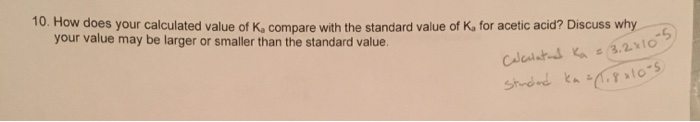This is from a Study of Buffer Solutions and pH of Salt Solutions Lab. I calculated Ka to be 3.2*10^-5. Why is my value larger than the standard value? Procedure: 10. How does your calculated value of Ka compare with the standard value of Ka for acetic acid? Discuss why your value may be larger or smaller than the standard value. Caleutats Ka 3.2x 10-5) Cyato-s Learning Objectives: 1. To test the acidic and basic properties of ionic compounds 2....

• ### I need to know if I calculated the acetate buffer correctly & how to calculate the...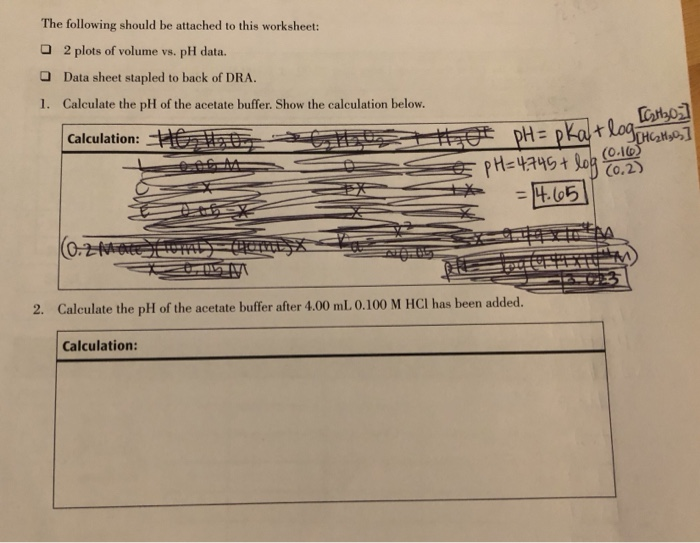I need to know if I calculated the acetate buffer correctly & how to calculate the change in pH when HCl is added... The following should be attached to this worksheet: 2 plots of volume vs. pH data. Data sheet stapled to back of DRA. 1. Calculate the pH of the acetate buffer. Show the calculation below. Calculation: Ho t t 419 [Cathoz ot pH= pkatlog HGH sos] Œ pH=4.745 + log NAH ** = 4.65 (0.2 m m 2....

• ### What is change in pH upon addition of 1.0x10-3mol strong acid to 1.00L of a buffer...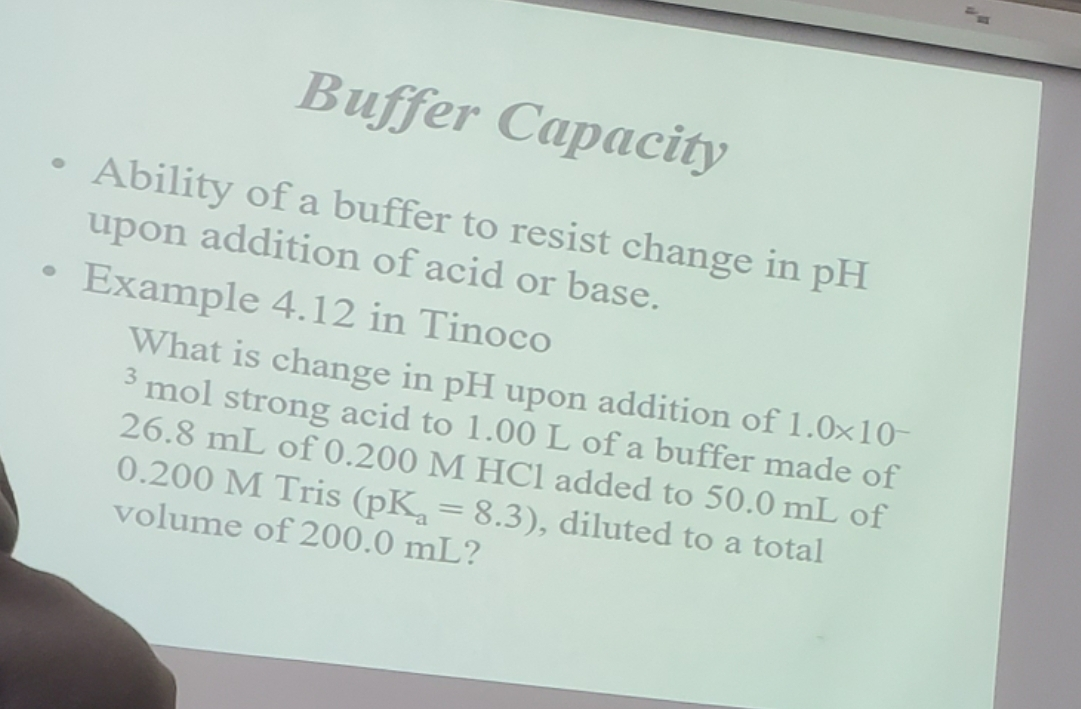What is change in pH upon addition of 1.0x10-3mol strong acid to 1.00L of a buffer mader of 26.8mL of 0.200M HCl added to 50.0mL of 0.200M Tris (pKa=8.3), diluted to a total volume of 0.200mL? Buffer Capacity • Ability of a buffer to resist change in pH upon addition of acid or base. Example 4.12 in Tinoco What is change in pH upon addition of 1.0x10- 3 mol strong acid to 1.00 L of a buffer made of 26.8...

• ### Tutored Practice Problem 17.2.5 COINS TOWARDS CRABE Calculate buffer pH after adding strong acid or strong base. Cl...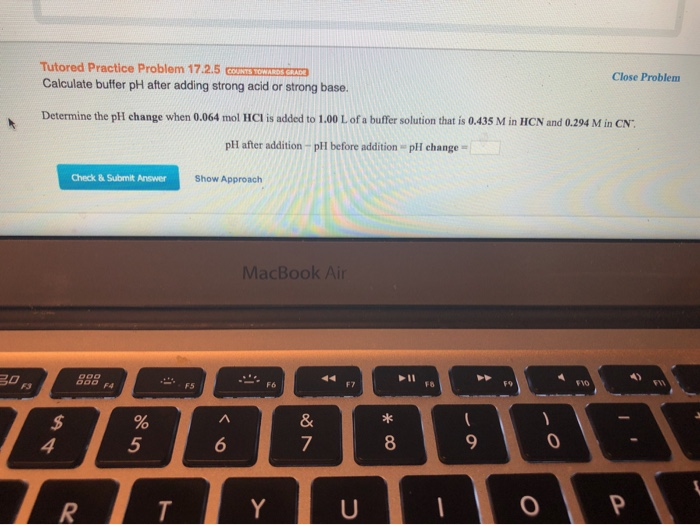Tutored Practice Problem 17.2.5 COINS TOWARDS CRABE Calculate buffer pH after adding strong acid or strong base. Close Problem Determine the pH change when 0.064 mol HCl is added to 1.00 L of a buffer solution that is 0.435 M in HCN and 0.294 M in CN pH after addition - pH before addition pH change - Check & Submit Answer Show Approach MacBook Air @0FBB U

• ### Please help with the prelab questions! thank you!!!! Especially 2 and 3! Pre-Lab Questions 1. Calculate...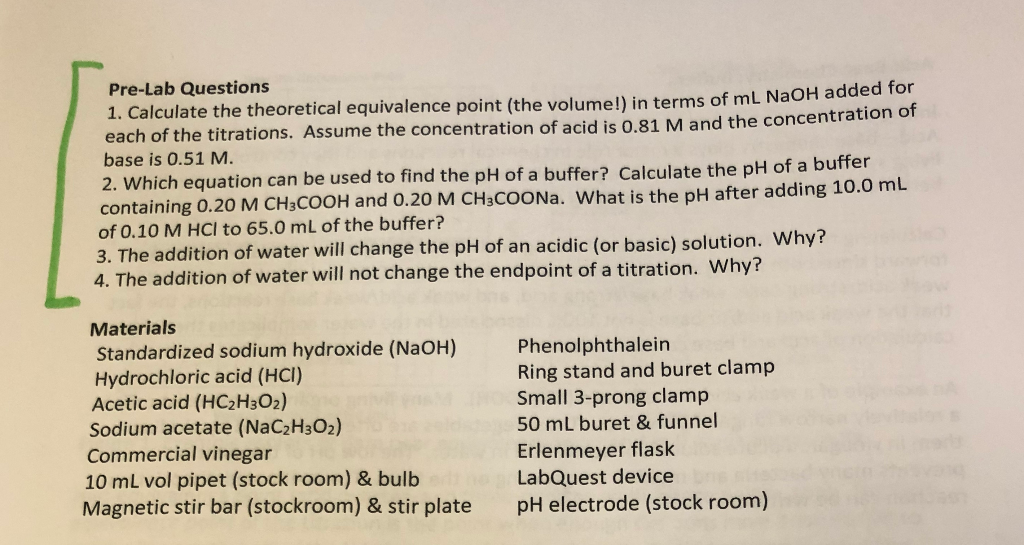Please help with the prelab questions! thank you!!!! Especially 2 and 3! Pre-Lab Questions 1. Calculate the theoretical equivalence point (the volume!) in terms of ml NaOH adde each of the titrations. Assume the concentration of acid is 0.81 M and the concentration of base is 0.51 M. 2. Which equation can be used to find the pH of a buffer? Calculate the pH of a buffer containing 0.20 M CH3COOH and 0.20 M CH3COONa. What is the pH after...

• ### How does a buffer resist change in pH upon addition of a strong acid? The strong...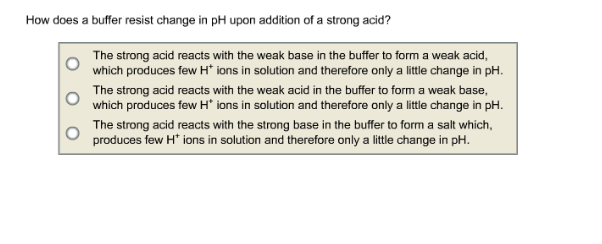How does a buffer resist change in pH upon addition of a strong acid? The strong acid reacts with the weak base in the buffer to form a weak acid, which produces few H + ions in solution and therefore only a little change in pH. The strong acid reacts with the weak acid in the buffer to form a weak base, which produces few H+ ions in solution and therefore only a little change in pH. The strong acid...

• ### For the most effective buffer solution for resisting PH change upon addition of strong base, determine...

For the most effective buffer solution for resisting PH change upon addition of strong base, determine the maximum amount of strong base it can absorb. Justify with calculation